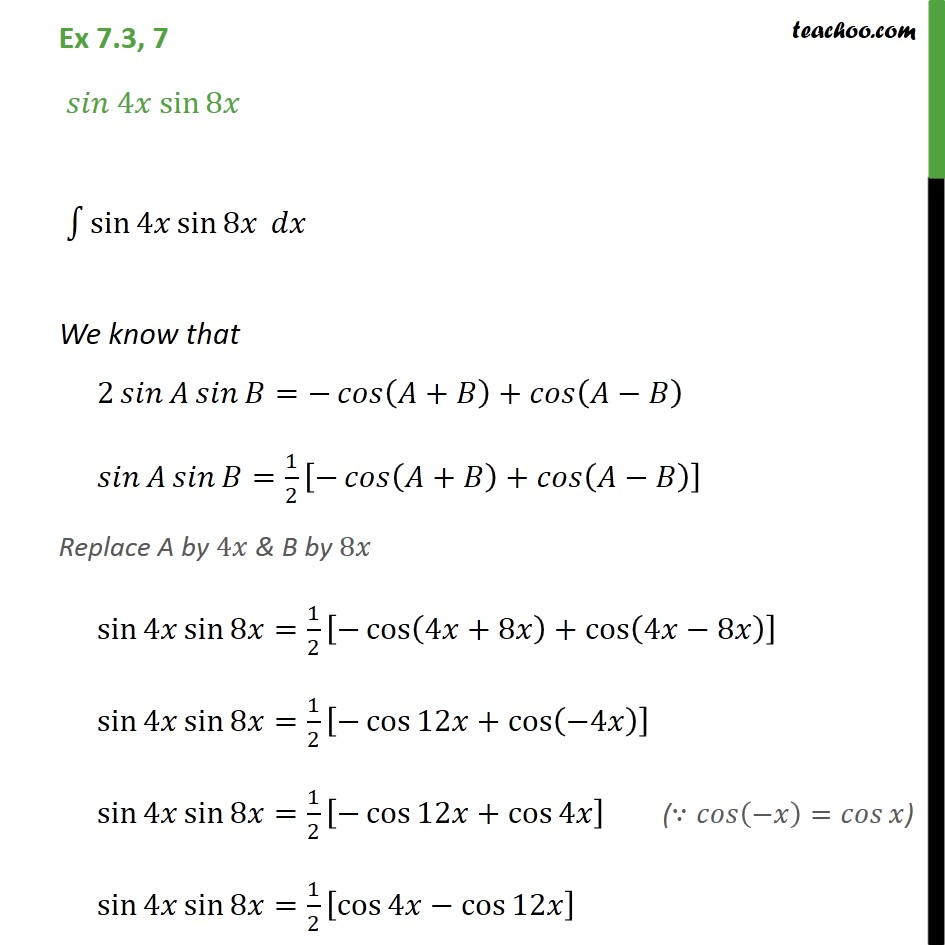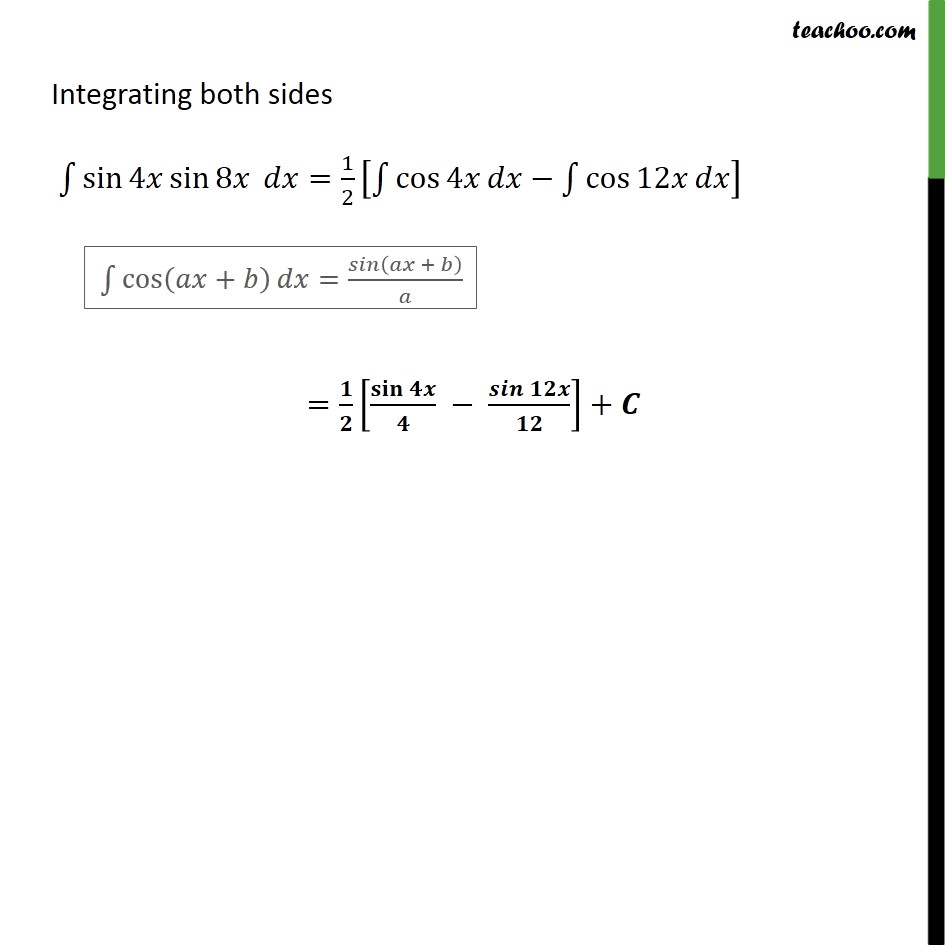Integration using trigo identities - CD and CD inv formulae

Chapter 7 Class 12 Integrals
Concept wiseLearn in your speed, with individual attention - Teachoo Maths 1-on-1 Class

### Transcript

Ex 7.3, 7 4 sin 8 sin 4 sin 8 We know that 2 = + + = 1 2 + + Replace A by 4 & B by 8 sin 4 sin 8 = 1 2 cos 4 +8 + cos 4 8 sin 4 sin 8 = 1 2 cos 12 + cos 4 sin 4 sin 8 = 1 2 cos 12 + cos 4 sin 4 sin 8 = 1 2 cos 4 cos 12 Integrating both sides sin 4 sin 8 = 1 2 cos 4 cos 12 = +